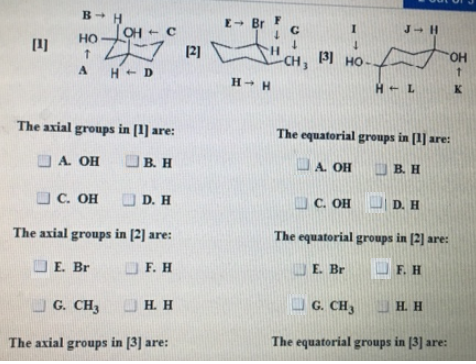# Problem: The axial groups in  are: The equatorial groups in  are: The axial groups in  are: The equatorial groups in  are: The axial groups in  are: The equatorial groups in  are:

###### FREE Expert Solution
94% (457 ratings)###### Problem Details

The axial groups in  are:

The equatorial groups in  are:

The axial groups in  are:

The equatorial groups in  are:

The axial groups in  are:

The equatorial groups in  are: